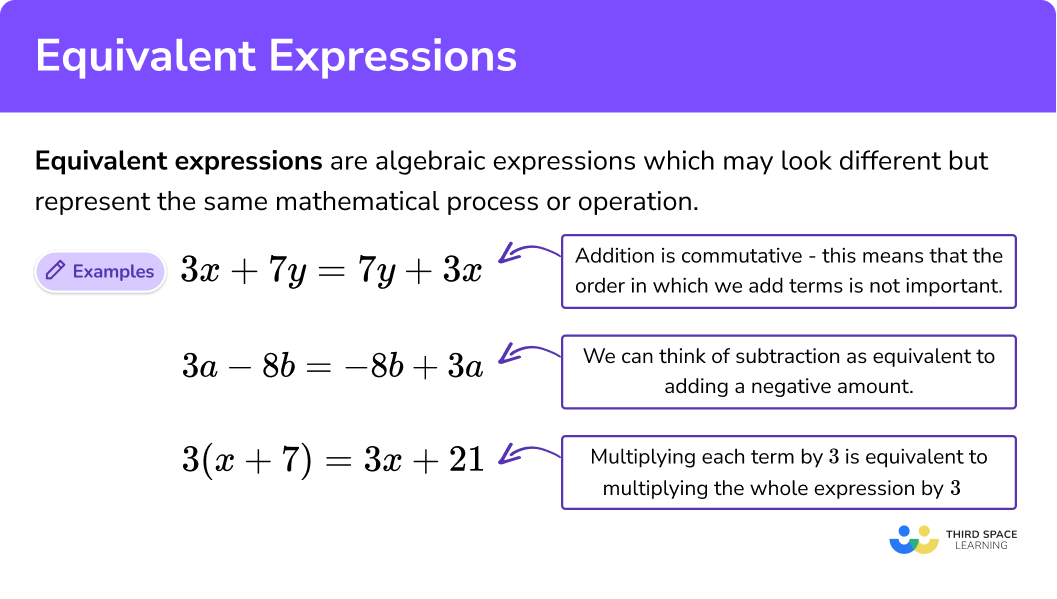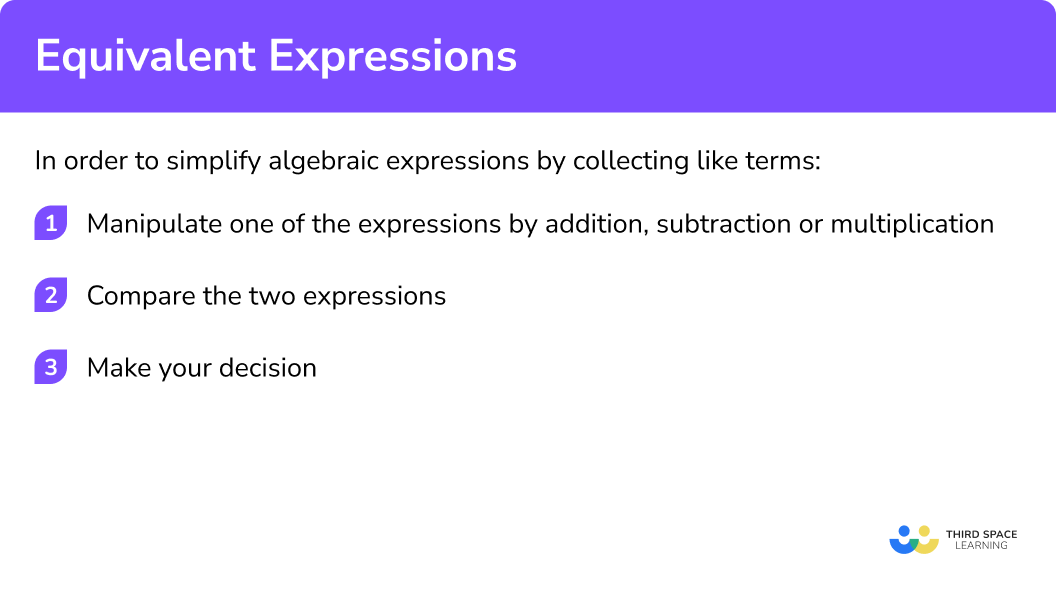GCSE Maths Algebra Algebraic Simplifying

Equivalent Expressions

# Equivalent Expressions

Here we will learn about equivalent expressions, including what they are and how to recognise them.

There are also equivalent expressions worksheets with practice problems based on Edexcel, AQA and OCR exam questions, along with further guidance on where to go next if you’re still stuck.

## What are equivalent expressions?

Equivalent expressions are algebraic expressions which may look different but represent the same mathematical process or operation.

An algebraic expression is two or more numbers and letters that are combined with mathematical operations such as \; +, \; -, \; \times \; or \; \div .

Two expressions are equivalent if they have the same value for any possible substituted value(s) of the variable(s) involved. We use the symbol \; \equiv \; to show that two expressions are equivalent.

For example, the equation 3x+2=14 is only true for one value of x, i.e. x=3.

But the statement 3x+2=2+3x is true for all possible values of x that we could substitute in, so we write 3x+2\equiv2+3x

Let’s look at some more examples of equivalent expressions.

### What are equivalent expressions?Addition is commutative which means the order in which we add the terms is not important – the answer will be the same.

For example, 3x+7y\equiv7y+3x.

3x+7y is the same as 7y+3x because the values of the variables match. By this we mean that the coefficients of x are the same, the coefficients of y are the same and they are added together. This means they are equivalent expressions.

For example, 3a-8b=3a+(-8b)\equiv-8b+3a.

The negative sign belongs to the term coming straight after it. It may be helpful to think of subtraction as adding a negative number.

3a-8b is the same as 3a+(-8b) which is the same as -8b+3a because when we remove the brackets (parentheses) we are a left with + \; - which is the same as -. This means they are equivalent expressions.

• Multiplication

Multiplication has a distributive property which allows us to break up more complicated multiplication calculations into two simpler parts.

For example, to calculate 3 \times 24 we can split the 24 into 20+4. We can then work out 3 \times 20 and 3 \times 4 and add them together.

So 3 \times 24=3 \times (20+4)=3 \times 20+3 \times 4=60+12=72.

This is used in algebra when we expand brackets.

For example, 3(x+7)\equiv3x+21.

3(x+7) is the same as 3x+21 because 3 \times x=3x and when we multiply the constants 3 and 7 we get 21. The right side is the same as the left side so they are equivalent expressions.

## How to use equivalent expressions

In order to use equivalent expressions:

1. Manipulate one of the expressions by addition, subtraction or multiplication.
2. Compare the two expressions.

### Explain how to use equivalent expressions## Equivalent expressions examples

### Example 1: addition and subtraction

Are the expressions 4a+7b and 7b-4a equivalent?

1. Manipulate one of the expressions by addition, subtraction or multiplication.

We can take the first expression and change the order of terms being added, 4a+7b becomes 7b+4a.

2Compare the two expressions.

The two expressions both have ‘4a’ and ‘7b’, but in the second expression the ‘4a’ is negative. It is ‘-4a’

The two expressions are not the same. They are NOT equivalent expressions.

### Example 2: addition and subtraction

Are the expressions 3c-8d and 8d-3c equivalent?

Manipulate one of the expressions by addition or subtraction or multiplication.

Compare the two expressions.

### Example 3: addition and subtraction

Are the expressions 6 p q^{2}+3 p and 3 p+6 p q^{2} equivalent?

Manipulate one of the expressions by addition or subtraction or multiplication.

Compare the two expressions.

### Example 4: expanding brackets and factorising

Are the expressions 4(x-5) and 4x-20 equivalent?

Manipulate one of the expressions by addition or subtraction or multiplication.

Compare the two expressions.

### Example 5: expanding brackets and factorising

Are the expressions p(3p+2) and 3p^{2}-2p equivalent?

Manipulate one of the expressions by addition or subtraction or multiplication.

Compare the two expressions.

### Example 6: expanding brackets and factorising

Are the following expressions equivalent?

-(y-5) and 5-y.

Manipulate one of the expressions by addition or subtraction or multiplication.

Compare the two expressions.

### Common misconceptions

• The order of subtraction is important

Remember that the order of a subtraction sum is very important.

7-4 4-7

\hspace{.23cm} + \; 3 - \; 3

• Confusing multiplying by \bf{2} and squaring

Remember that x+x is written as 2x. This means 2 \times x, or 2 lots of x.

Remember that x multiplied by itself is x \times x which is written as x^{2}.

2x and x^{2} are two different types of algebraic terms.

• Algebraic terms with \bf{2} or more letters

Remember that algebraic terms involving 2 or more letters tend to be written with the letters in alphabetical order. a \times c \times b tends to be written as abc rather than acb.

### Practice equivalent expressions questions

1. Which is the equivalent expression to 4+5x ?

4x+55+4x5x+44-5xThe original expression can be changed so that the order in which the two terms are added is reversed.

4+5x\equiv5x+4

2. Which is the equivalent expression to 3a-4b ?

3a+4b-3a+4b4b-3a-4b+3aThe original expression can be changed so that the order in which the two terms are added is reversed.

3a-4b=3+(-4b)\equiv-4b+3a

3. Which is the equivalent expression to 5x^2+6y^3 ?

6y^2+5x^36y^3+5x^26x^2+5y^36x^3+5y^2The original expression can be changed so that the order in which the two terms are added is reversed.

5x^2+6y^3\equiv6y^3+5x^2

4. Which is the equivalent expression to 3(a+4) ?

3a-123a-43a+43a+12The original expression can be expanded.

3(a+4)=3 \times a+3 \times 4\equiv3a+12

5. Which is the equivalent expression to -(x-8) ?

8-x-8-x8+x-x-8The original expression can be expanded.

-(x-8)=-1(x-8)=-1\times x + -1\times -8=-x+8\equiv8-x

6. Which is the equivalent expression to a(a+3) ?

a^2+3a^2-3aa^2+3a2a+a^3The original expression can be expanded.

a(a+3)=a \times a+3 \times a\equiv a^{2}+3a

### Equivalent expressions GCSE questions

1. Circle the equivalent expression to 3x^{2}-5y^{3}.

-3x^{2}+5y^{3} \hspace{1cm} -5y^{3}+3x^{2} \hspace{1cm} 3y^{2}-5x^{2} \hspace{1cm} -5y^{2}+3x^{2}

(1 mark)

-5y^{3}+3x^{2}

(1)

2. Circle the equivalent expression to x(2x-5).

x^{3}-5x \hspace{1cm} x^{2}-5x \hspace{1cm} 2x^{2}-5 \hspace{1cm} 2x^{2}-5x

(1 mark)

2x^{2}-5x

(1)

3. Match the equivalent expressions.

a+a+b+b+b \hspace{3cm} 2a-ab \\ 2a+4b-a-2b \hspace{3cm} a^{2}-ab \\ (a+b)(a-b) \hspace{3.4cm} 2a+3b \\ a(2-b) \hspace{4.2cm} 4b-2a \\ a(a-b) \hspace{4.2cm} 2a-2b \\ -2a+4b \hspace{4.1cm} a+2b \\ 2(a-b) \hspace{4.2cm} a^{2}-b^{2} \\

(3 marks)

a+a+b+b+b \hspace{3cm} 2a+3b

2a+4b-a-2b \hspace{3cm} a+2b

(a+b)(a-b) \hspace{3.4cm} a^{2}-b^{2}

a(2-b) \hspace{4.1cm} 2a-ab

a(a-b) \hspace{4.1cm} a^{2}-ab

-2a+4b \hspace{4cm} 4b-2a

2(a-b) \hspace{4.1cm} 2a-2b

(3)

## Learning checklist

You have now learned how to:

• Recognise equivalent expressions

## Still stuck?

Prepare your KS4 students for maths GCSEs success with Third Space Learning. Weekly online one to one GCSE maths revision lessons delivered by expert maths tutors.

Find out more about our GCSE maths tuition programme.